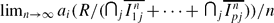Hostname: page-component-594f858ff7-7tp2g Total loading time: 0 Render date: 2023-06-08T11:35:54.724Z Has data issue: false Feature Flags: { "corePageComponentGetUserInfoFromSharedSession": true, "coreDisableEcommerce": false, "corePageComponentUseShareaholicInsteadOfAddThis": true, "coreDisableSocialShare": false, "useRatesEcommerce": true } hasContentIssue false

# Partial Castelnuovo–Mumford regularities of sums and intersections of powers of monomial ideals

Published online by Cambridge University Press:  16 March 2010

## Abstract

Let I, I11,. . ., I1q1,. . ., Ip1,. . ., Ipqp be monomial ideals of a polynomial ring R = K[X1,. . ., Xr] and Ln = I+∩jIn1j + ⋅ ⋅ ⋅ + ∩jIpjn. It is shown that the ai-invariant ai(R/Ln) is asymptotically a quasi-linear function of n for all n ≫ 0, and the limit limn→∞ad(R/Ln)/n exists, where d = dim(R/L1). A similar result holds if I11,. . ., I1q1,. . ., Ip1,. . ., Ipqp are replaced by their integral closures. Moreover all limitsalso exist.

As a consequence, it is shown that there are integers p > 0 and 0 ≤ ed = dim R/I such that reg(In) = pn + e for all n ≫ 0 and pn ≤ reg(In) ≤ pn + d for all n > 0 and that the asymptotic behavior of the Castelnuovo–Mumford regularity of ordinary symbolic powers of a square-free monomial ideal is very close to a linear function.

Type
Research Article
Information

## Access options

Get access to the full version of this content by using one of the access options below. (Log in options will check for institutional or personal access. Content may require purchase if you do not have access.)

## References

#### REFERENCES

Cutkosky, D., Ein, L. and Lazarsfeld, R.Positivity and complexity of ideal sheaves. Math. Ann. 321 (2001), no. 2, 213234.CrossRefGoogle Scholar
Cutkosky, D., Herzog, J., Trung, N. V.Asymptotic behavior of the Castelnuovo–Mumford regularity. Compositio Math. 118 (1999), 243261.CrossRefGoogle Scholar
Eisenbud, D.Commutative algebra with a view toward algebraic geometry. Graduate Texts in Math. (Springer-Verlag, 1995).Google Scholar
Giang, D. H. and Hoa, L. T. On local cohomology of a tetrahedral curve. Acta Math. Vietnam. (to appear); ArXiv: 0910.0919.Google Scholar
Goto, S. and Watanabe, K.On graded rings, I. J. Math. Soc. Japan. 30 (1978), 179213.CrossRefGoogle Scholar
Herzog, J., Hibi, T. and Trung, N. V.Symbolic powers of monomial ideals and vertex cover algebra. Adv. Math. 210 (2007), 304322.CrossRefGoogle Scholar
Herzog, J., Hoa, L. T. and Trung, N. V.Asymptotic linear bounds for the Castelnuovo–Mumford regularity. Trans. Amer. Math. Soc. 354 (2002), 17931809.CrossRefGoogle Scholar
Hoa, L. T. and Hyry, E.On local cohomology and Hilbert function of powers of ideals. Manuscripta Math. 112 (2003), 7792.CrossRefGoogle Scholar
Hoa, L. T. and Trung, N. V.On the Castelnuovo–Mumford regularity and the arithmetic degree of monomial ideals. Math. Z. 229 (1998), 519537.CrossRefGoogle Scholar
Hoa, L. T. and Trung, T. N.Castelnuovo–Mumford regularity of sums of powers of polynomial ideals. Comm. Algebra 36 (2008), 806820.CrossRefGoogle Scholar
Katzman, M.The complexity of Frobenius powers of ideals. J. Algebra 203 (1998), 211225.CrossRefGoogle Scholar
Kodiyalam, V.Asymptotic behavior of Castelnouvo-Mumford regularity. Proc. Amer. Math. Soc. 128 (2000), 407411.CrossRefGoogle Scholar
Reid, L., Roberts, L. G. and Vitulli, M. A.Some results on normal homogeneous ideals. Comm. Algebra 31 (2003), 44854506.CrossRefGoogle Scholar
Schrijver, A., Theory of linear and integer programming. (John Wiley & Sons, Ltd., 1986).Google Scholar
Stanley, R. P.Combinatorics and Commutative Algebra (Birkhauser, 1996).Google Scholar
Takayama, Y.Combinatorial characterizations of generalized Cohen-Macaulay monomial ideals. Bull. Math. Soc. Sci. Math. Roumanie (N. S.) 48 (96) (1987), 327344.Google Scholar
Trung, N. V.Gröbner bases, local cohomology and reduction number. Proc. Amer. Math. Soc. 129 (2001), 918.CrossRefGoogle Scholar
Trung, N. V. and Wang, H. J.On the asymptotic linearity of Castelnuovo–Mumford regularity. J. Pure Appl. Algebra 201 (2005), 4248.CrossRefGoogle Scholar
Trung, T. N.Regularity index of Hilbert function of powers of ideals. Proc. Amer. Math. Soc. 137 (2009), 21692174.CrossRefGoogle Scholar